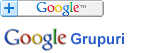### Căutare în Webster - Dicționarul explicativ al limbii engleze

 Cuvânt
Pentru căutare rapidă introduceți minim 3 litere.

## LOGARITHM - Definiția din dicționar

Traducere: română

Notă: Puteţi căuta fiecare cuvânt din cadrul definiţiei printr-un simplu click pe cuvântul dorit.

Log"a*rithm (lŏg"&adot_;*rĭ&thlig_;'m), n. [Gr. lo`gos word, account, proportion + 'ariqmo`s number: cf. F. logarithme.] (Math.)

`0    1    2     3      4       Indices or logarithms1   10   100  1000  10,000     Numbers in geometrical progression`

Hence, the logarithm of any given number is the exponent of a power to which another given invariable number, called the base, must be raised in order to produce that given number. Thus, let 10 be the base, then 2 is the logarithm of 100, because 102 = 100, and 3 is the logarithm of 1,000, because 103 = 1,000.
[1913 Webster]

Arithmetical complement of a logarithm, the difference between a logarithm and the number ten. -- Binary logarithms. See under Binary. -- Common logarithms, or Brigg's logarithms, logarithms of which the base is 10; -- so called from Henry Briggs, who invented them. -- Gauss's logarithms, tables of logarithms constructed for facilitating the operation of finding the logarithm of the sum of difference of two quantities from the logarithms of the quantities, one entry of those tables and two additions or subtractions answering the purpose of three entries of the common tables and one addition or subtraction. They were suggested by the celebrated German mathematician Karl Friedrich Gauss (died in 1855), and are of great service in many astronomical computations. -- Hyperbolic logarithm or Napierian logarithm or Natural logarithm, a logarithm (devised by John Speidell, 1619) of which the base is e (2.718281828459045...); -- so called from Napier, the inventor of logarithms. -- Logistic logarithms or Proportional logarithms, See under Logistic.
[1913 Webster]